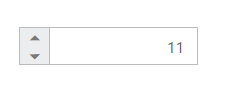# RTL Support

The NumericTextBox provides RTL (Right-To-Left) support. The alignment of NumericTextBox can be changed from Left-To-Right into Right-To-Left.

## Enable RTL

The following steps explain the implementation of enableRTL in NumericTextBox .

In the HTML page set the corresponding <input> elements for rendering NumericTextBox control.

• html
• ``<input id="numeric" type="text" ej-numerictextbox e-value="11" e-enablertl="true" />``

The output for NumericTextBox when enableRTL is “true” is as follows.